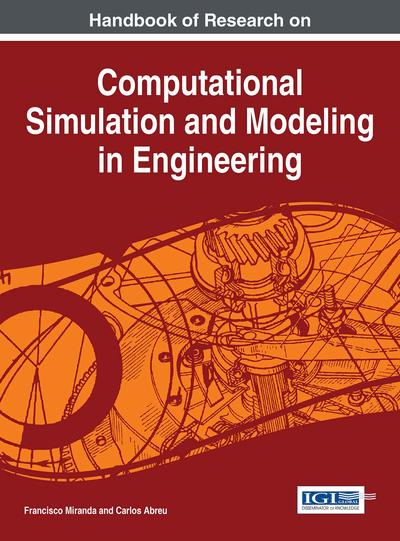# Energy Conservation Law for the Turbulent Motion in the Free Atmosphere: Turbulent Motion in the Free Atmosphere

Kulyash Kaliyeva (Lorraine University, France)
DOI: 10.4018/978-1-4666-8823-0.ch003

## Abstract

This chapter presents convergent-divergent flow in the free atmosphere which is governed by the three dimensional Navier-Stokes equations and deals with the fundamental problem of fluid dynamics. Considering air movement under influence divergence and rotation were found the true dependencies between the velocity vector and the pressure distribution. Following the classical procedure by using rotor operator and a well-known formula of vector analysis were obtained the second kind nonlinear Volterra-Fredholm integral equations in a matrix form which contained only three components of the velocity vector. According to the theory of the matrix operators were defined the velocity components by the successive approximation method. According to the obtained balance equation for the pressure distribution were defined significant properties of the transient convergent-divergent flow which provide a description of the constitutive relationships between three physical quantities: the velocity vector, the external and internal forces, the pressure distribution.
Chapter Preview
Top

## Introduction

Theoretical prediction and practical analysis of turbulence are the fundamental problems of atmospheric dynamics. Turbulence is considered as an eddy motion with a wide spectrum of sizes. Turbulent motion is a nonlinear multistage phenomenon of the elusive process which under influence divergence and rotation is characterized by high Reynolds numbers and involves some difficult problems in theoretical and mathematical physics. Due to the mathematical theory of partial differential equations introduced turbulent models were based on the transport equation of the most appropriate convergent-divergent motions. The Navier-Stokes equations are described interactions between fluctuations and their space-time directions of the different wavelengths which have a great theoretical interest and practical importance in mathematical and physical modeling of elusive atmospheric phenomena of turbulence. Here were found dependencies of the velocity vector from the pressure distribution which make varying turbulent effects in agreement with the energy conservation law. It was shown the space-time dependent impact of divergence of the velocity vector and the density. Considering a flow element at a space-time position has been found energy balance which is expressed relationship between three physical quantities: the velocity vector, the external and internal forces, the pressure distribution. Balance equation expresses the second law of thermodynamics, which says that in all natural world processes of the entropy always increases. Obtained universal energy balance equation in the principal form expresses a stability condition which is an important part in simplifying the processing and analysis of the theoretical and experimental date of the turbulent field problems. It was shown that in the particular case of the energy balance equation can be got Bernoulli’s equation. Atmospheric flow is considered by the Navier-Stokes equations in agreement with the second law of thermodynamics where external forcing is supplied by radioactive forcing from the Sun, Lorentz force, dynamic Carioles force, gravity and acceleration due to the rotating Earth and heat sources at ground. The language and methodology of this research is the mathematical theory of partial differential equations. We have focused on the global existence, uniqueness and smoothness of weak solution of the Navier-Stokes equations. Investigation of the analytical solvability for a practical complex problem in infinite space for the nonlinear turbulent phenomenon requires a perspective using some alternative approach which different from that is needed for studying the general classical issues in determining the influence of divergence and rotation from the turbulent velocity vector. Following the classical procedure by using rotor operator and a well-known formula of vector analysis were obtained the second kind nonlinear Volterra-Fredholm integral equations in a matrix form which contained only three components of the velocity vector. According to the theory of the Volterra-Fredholm matrix operators were defined the velocity components by the successive approximation method. Considering the pressure gradient as a potential field was determined the pressure distribution which satisfies the energy conservation law. We have clear mathematical evidence that the conservative force fields effectively make fluctuations in infinite space. Thank to the defined estimations for the velocity vector and the pressure distribution were proved existence and uniqueness theorems in the potential stable fields and general cases of bifurcating instabilities.

## Key Terms in this Chapter

Divergence: A meteorological condition characterized by the uniform expansion in volume of a mass of air over a region, usually accompanied by fair dry weather

Turbulence: Instability in the atmosphere

Physical Phenomenon: A natural phenomenon involving the physical properties of matter and energy

Irregular Motion of the Atmosphere: As that indicated by gusts and lulls in the wind.

Dynamic Viscosity: The shear stress applied divided by the velocity gradient achieved when a shear force is applied to a fluid

Incompressible Flow: Flow when the density is constant.

Energy Conservation: The fundamental principle of physics that the total energy of an isolated system is constant despite internal changes

Compressible Flow: Flow when the density is not constant.

Rotation: The movement or path of the earth or a heavenly body turning on its axis.

Kinematic Viscosity: A measure of the resistive flow of a fluid under the influence of gravity.

## Complete Chapter List

Search this Book:
Reset